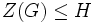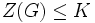# Local powering-invariant subgroup containing the center is intermediately local powering-invariant in nilpotent group

## Statement

Suppose$G$ is a nilpotent group and$H$ is a subgroup containing the center of$G$ that is also a local powering-invariant subgroup of$G$. Then,$H$ is an intermediately local powering-invariant subgroup of$G$. Explicitly, suppose$K$ is a subgroup of$G$ containing$H$. Then,$H$ is a local powering-invariant subgroup of$K$.

## Facts used

1. Torsion-freeness for a prime is subgroup-closed
2. Equivalence of definitions of nilpotent group that is torsion-free for a set of primes
3. Nilpotency is subgroup-closed

## Proof

Given: A nilpotent group$G$, a subgroup$H$ of$G$ that is local powering-invariant and such that$Z(G) \le H$ where$Z(G)$ is the center of$G$. A subgroup$K$ of$G$ containing$H$. A prime number$p$ and an element$h \in H$ such that there is a unique element$x \in K$ satisfying$x^p = h$.

To prove: There exists a unique element$x \in H$ such that$x^p = h$.

Proof:

Step no. Assertion/construction Facts used Given data used Previous steps used Explanation
1$Z(G) \le K$.$Z(G) \le H, H \le K$. given-direct
2$K$ is nilpotent. Fact (3)$G$ is nilpotent,$K \le G$ Given-fact direct
3$K$ is$p$-torsion-free. Fact (2)$h \in K$ has a unique$p^{th}$ root in$K$. We use the equivalence (3) implies (1) within the multi-part equivalence of Fact (2).
4$Z(G)$ is$p$-torsion-free. Fact (1) Steps (1), (3) Step-fact combination direct
5 The map$t \mapsto t^p$ is injective in$G$. Fact (2)$G$ is nilpotent Step (4) Step-fact combination direct (specifically, we want to use the implication from (4) to (1) in the multi-part equivalence of Fact (2))
6 The element$x \in K$is the unique$p^{th}$ root of$h$ in all of$G$.$x \in K$ satisfies$x^p = h$. Step (5) given-step direct
7 The element$x$ of Step (6) is in$H$.$H$ is local powering-invariant in$G$. Step (6) Step-given combination direct.